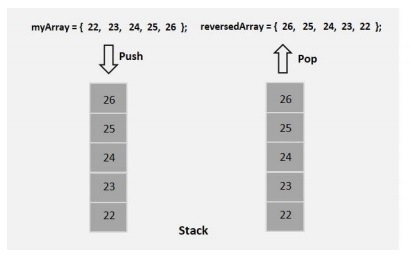# Java program to reverse an array

Stack is an Abstract Data Type (ADT), commonly used in most programming languages. It is named stack as it behaves like a real-world stack, for example – a deck of cards or a pile of plates, etc.

A stack is first in first out, it has two main operations push and pop. Push inserts data into it and pop retrieves data from it.

To reverse an array using stack initially push all elements into the stack using the push() method then, retrieve them back using the pop() method into another array.## Example

import java.util.Arrays;
import java.util.Stack;
public class ab38_ReverseOfArray {
public static void main(String args[]) {
Stack<Integer> stack = new Stack<Integer>();
int[] myArray = {23, 93, 56, 92, 39};
int size = myArray.length;
for(int i=0; i<size; i++) {
stack.push(myArray[i]);
}
int[] reverseArray = new int[size];
for(int i=0; i<size; i++) {
reverseArray[i] = stack.pop();
}
System.out.println("Reversed array is ::"+Arrays.toString(reverseArray));
}
}

## Output

Reversed array is ::[39, 92, 56, 93, 23]